# Electronics and Communication Engineering - Exam Questions Papers

21.

A Hartley oscillator uses FET with gm of 2 ms and rd = 2 KΩ. The total coil inductance is 10 μH with turns ratio of input to output side of 1 : 10. It is tuned with a 50 pf capacitor. Find frequency of oscillations, amplifier gain margin in dB.

 A. 7.11 MHz, 13.97 dB B. 7.3 MHz, 14.1 dB C. 6.93 MHz, 12.9 dB D. 7.4 MHz, 13 dB

Explanation: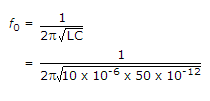= 7.11 MHz

μ = gm x rd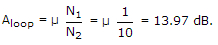22.

The recursion relation to solve x = e-x using Newton Raphson method is

 A. Xn+1 = e-xn B. Xn+1 = Xn - e-xn C.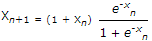D.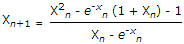Explanation:

f(xn) = xne-xn

f(xn) = 1 + e-xn f'(xn) = 1 + e-xn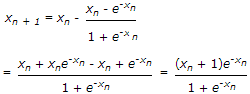23.

In the circuit shown in the given figure the diode states at the extremely large negative values of the input voltage vi are :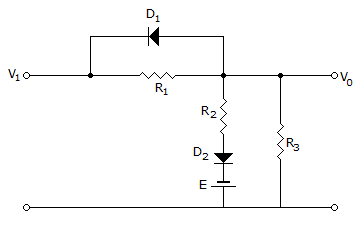A. D1 off, D2 off B. D1 on, D2 off C. D1 off, D2 on D. D1 on, D1 on, D2 on

Explanation:

Input V1 is extremely large negative value, so D1 is ON.

Diode D2 is shunted by R3 and the junction of R2R3 is more negative than the lower end.

Diode D2's cathode is at negative potential.

But the anode of D2 is less negative than E as the input voltage is extremely negative and D2 is OFF.

24.

Consider a baseband binary PAM receiver shown below. The additive channel noise n(t) is whit with power spectral density SN(f) = N0/2 = 10-20 W/Hz. The low-pass filter is ideal with unity gain and cutoff frequency 1 MHz. Let Yk represent the random variable y(tk).
Yk = nNk if transmitted bit bk = 0
Yk = a + Nk if transmitted bit bk = 1
Where Nk represents the noise sample value. The noise sample has a probability density function, PNk(n) = 0.5ae-a|n| (This has mean zero and variance 2/a2). Assume transmitted bits to be equiprobable and threshold z is set to a/2 = 10-6 V.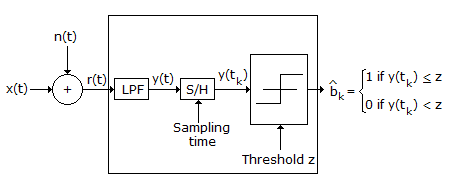The value of the parameter a (in V-1) is

 A. 1010 B. 107 C. 1.414 x 10-10 D. 2 x 10-20

Explanation: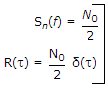→ exist in fourier transform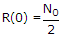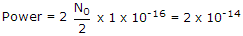Variance =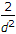which is equal to power since mean is zero ⇒ a = 107.

25.

The probability density function of a random variable x is as shown.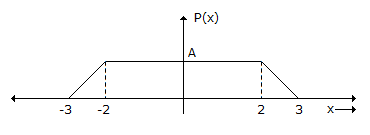The mean of the distribution is:

 A.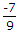B.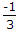C.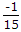D.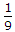Explanation:

Mean of the distribution =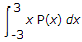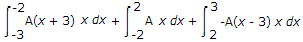A = 1/5 then find mean.

#### Current Affairs 2022

Interview Questions and Answers